4.9.1 Traversal by Recursion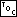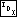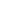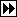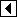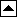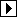To figure out how to traverse a binary search tree in inorder, think about a BST's structure. A BST consists of a root, a left subtree, and right subtree. All the items in the left subtree have smaller values than the root and all the items in the right subtree have larger values than the root.

That's good enough right there: we can traverse a BST in inorder by dealing with its left subtree, then doing with the root whatever it is we want to do with each node in the tree (generically, visit (see visit) the root node), then dealing with its right subtree. But how do we deal with the subtrees? Well, they're BSTs too, so we can do the same thing: traverse its left subtree, then visit its root, then traverse its right subtree, and so on. Eventually the process terminates because at some point the subtrees are null pointers, and nothing needs to be done to traverse an empty tree.

Writing the traversal function is almost trivial. We use bst_item_func to visit a node (see Item and Copy Functions):

```49. <Recursive traversal of BST 49> =
static void traverse_recursive (struct bst_node *node, bst_item_func *action, void *param) {
if (node != NULL)     {
traverse_recursive (node->bst_link, action, param);
action (node->bst_data, param);
traverse_recursive (node->bst_link, action, param);
}
}
```

We also want a wrapper function to insulate callers from the existence of individual tree nodes:

```50. <Recursive traversal of BST 49> +=
void walk (struct bst_table *tree, bst_item_func *action, void *param) {
assert (tree != NULL && action != NULL);
traverse_recursive (tree->bst_root, action, param);
}
```

See also:  [Knuth 1997], section 2.3.1; [Cormen 1990], section 13.1; [Sedgewick 1998], program 12.8.

Exercises:

1. Instead of checking for a null node at the top of traverse_recursive(), would it be better to check before calling in each place that the function is called? Why or why not? [answer]

2. Some languages, such as Pascal, support the concept of nested functions, that is, functions within functions, but C does not. Some algorithms, including recursive tree traversal, can be expressed much more naturally with this feature. Rewrite walk(), in a hypothetical C-like language that supports nested functions, as a function that calls an inner, recursively defined function. The nested function should only take a single parameter. (The GNU C compiler supports nested functions as a language extension, so you may want to use it to check your code.) [answer]